# Trigonometric Ratios of Complementary Angle

### Share solution sheet

1. Let us evaluate: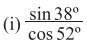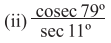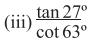2. Let us show that: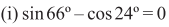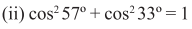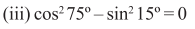2.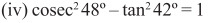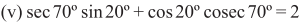3. If two angles α and β are complementary angles, let us show that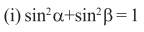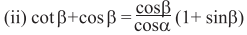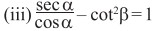4. If sin 17o = x/y, let us show that ;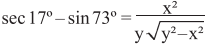5. let us show that;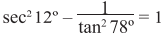6. ∠A + B =90o, let us show that ;

7. let us show that ;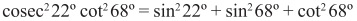8. If P + Q = 90o, let us show that ;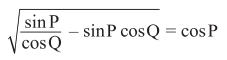9. let us prove that ;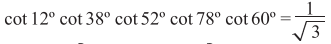10. AOB is a diameter of a circle with center O and C is any point on the circle, joining A.C, B,C and O,C let us show that;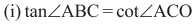10.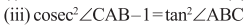11. ABCD is a rectangular figure, joining A, C let us prove that.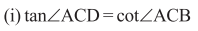## Related Posts

### Right Circular Cone

Q.1 I have made a closed right circular cone whose length of the base radius is 15 cm and slant height is 24 cm. Let

### Trigonometric Ratios and Trigonometric Identities

Exercise 23.1 1. I have drawn a right-angled triangled ABC whose hypotenuse AB=10 cm, base BC=8 cm. and perpendicular AC=6cm. Let us determine the values

### Rectangular Parallelopiped or Cuboid

3. The length, breadth, and height of a cuboidal Room are 5 m, 4 m, and 3m respectively, let us write the length of the

### Trigonometry : Concept of Measurement of Angle

Exercise 20 Let us express the following into degrees, minutes, and seconds. (i) 832’     (ii) 6312”    (iii) 375”(iv) 27 (1/12)o    (v) 72.04o